# Standard 4 Gases Chemistry Ms Siddall Standard 4

• Slides: 45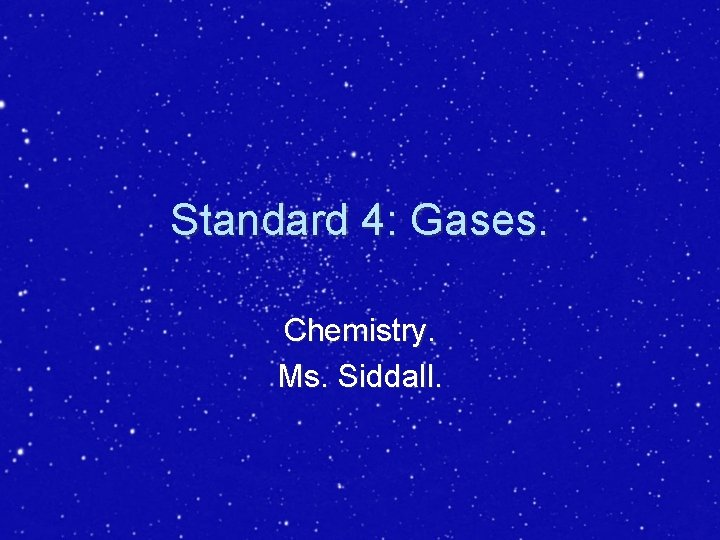Standard 4: Gases. Chemistry. Ms. Siddall.Standard 4 f: Lowest Possible Temperature Absolute zero = temperature where there is no particle movement (no kinetic energy). ØThere can be no lower temperature. Øabsolute zero = 0 Kelvin = 0 K ØDeep space ~ 2. 7 K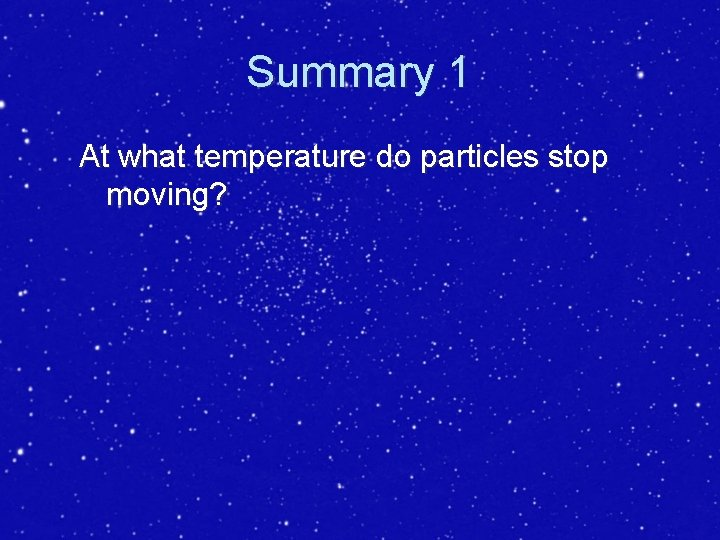Summary 1 At what temperature do particles stop moving?Standard 4 e: Kelvin/Celsius conversions Kelvin temperature = °C + 273 § Example: room temperature is about 22°C. What is that in Kelvin? Kelvin = °C + 273 = 22 + 273 = 295 K § Example: What is 0 K in °C? °C = Kelvin - 273 = 0 - 273 = -273°C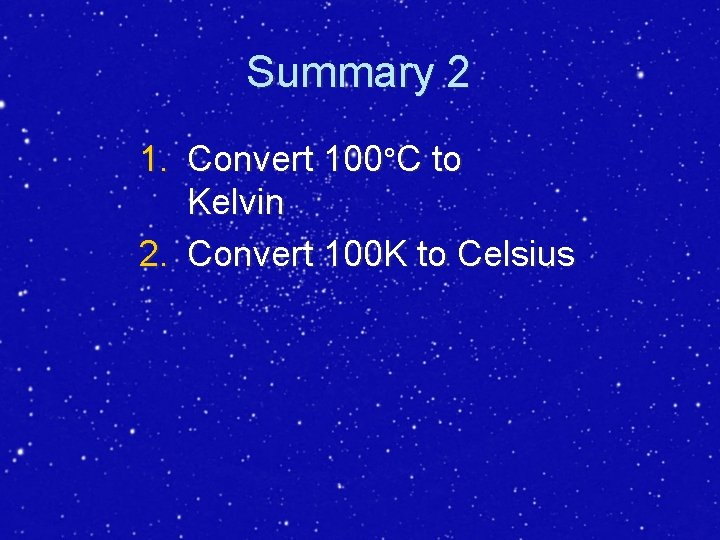Summary 2 1. Convert 100°C to Kelvin 2. Convert 100 K to CelsiusStandard 4 d: Standard Temperature and Pressure STP: 1 mole of gas = 22. 4 L Temperature = 0°C = 273 K Pressure = 1 atm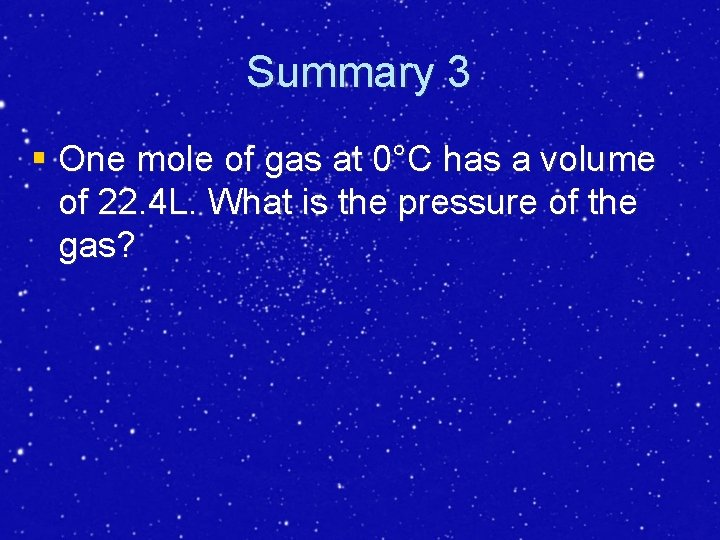Summary 3 § One mole of gas at 0°C has a volume of 22. 4 L. What is the pressure of the gas?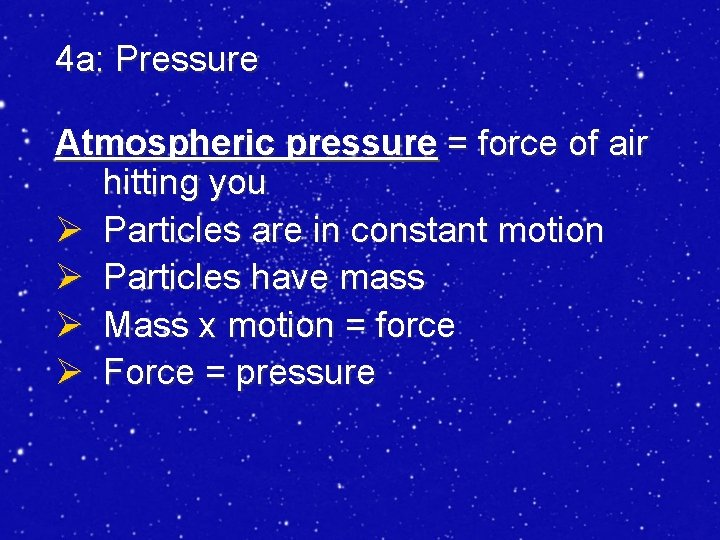4 a: Pressure Atmospheric pressure = force of air hitting you Ø Particles are in constant motion Ø Particles have mass Ø Mass x motion = force Ø Force = pressure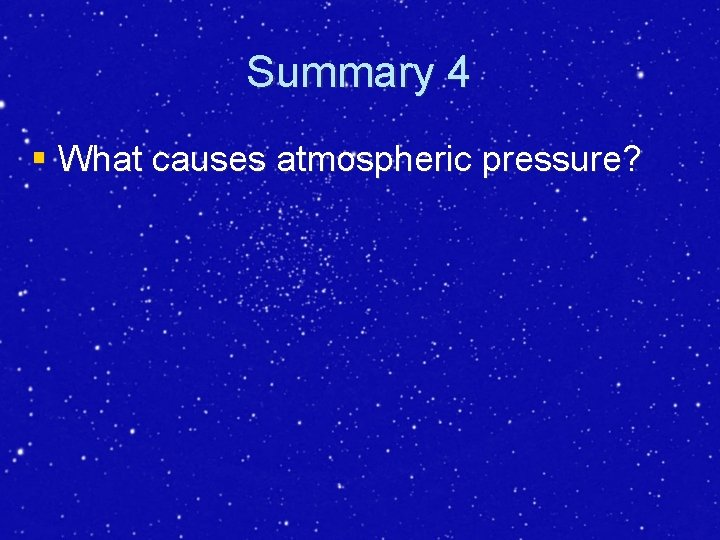Summary 4 § What causes atmospheric pressure?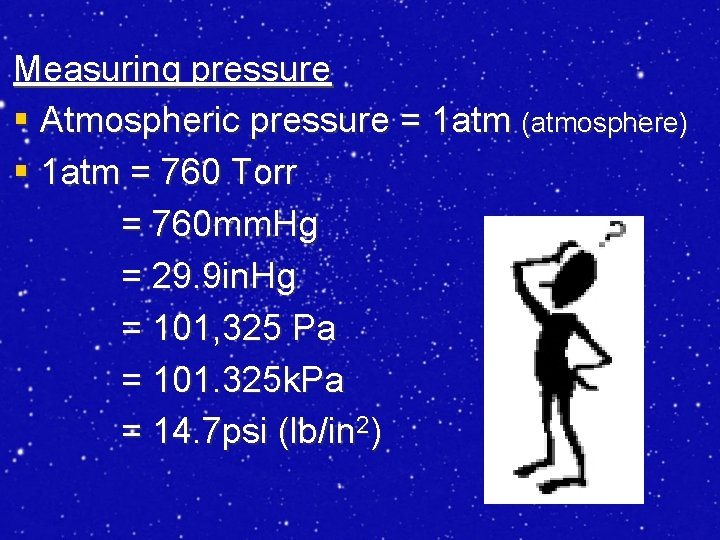Measuring pressure § Atmospheric pressure = 1 atm (atmosphere) § 1 atm = 760 Torr = 760 mm. Hg = 29. 9 in. Hg = 101, 325 Pa = 101. 325 k. Pa = 14. 7 psi (lb/in 2)§ Example: Coca-Cola© is bottled at approximately 2 atmospheres. Convert to Torr. 2 atm 760 Torr 1 atm ~ 1520 TorrSummary 5 Convert 2 atm to: 1. Psi 2. kpaLiquids and gases compared • Atmospheric pressure = 14. 7 psi • Deep ocean pressure = 16, 000 psi • Water molecules are close together (high pressure) and air particles are far apart (lower pressure) • Density of water = 1 g/ml = 1000 g/L • Density of air = 1 g/L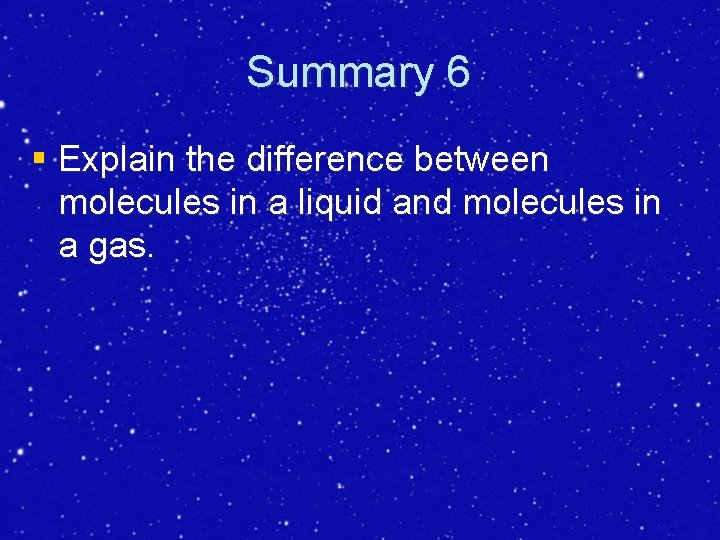Summary 6 § Explain the difference between molecules in a liquid and molecules in a gas.Kinetic Molecular Theory: 1. Gases consist of small particles that have mass. 2. The volume of a gas is mostly empty space. 3. There are no intermolecular attractions between gas particles. 4. Particles are in constant, random, rapid, straight line motion. 5. Collisions are elastic (no loss of energy). 6. Kinetic energy of particles depends on temperature.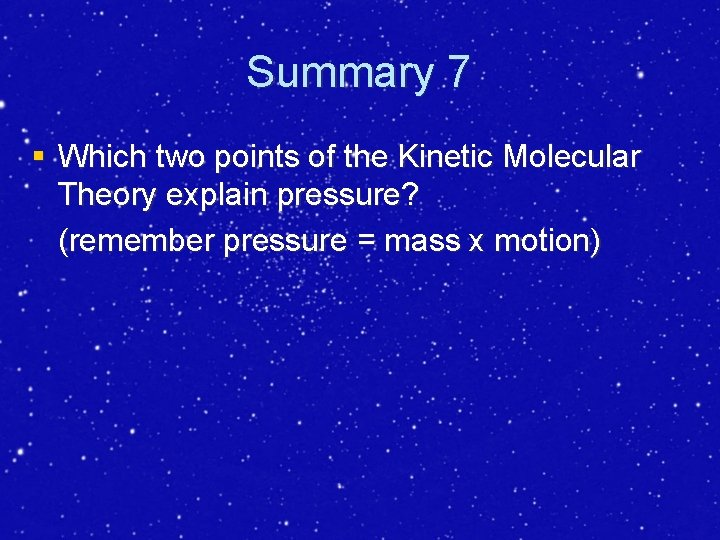Summary 7 § Which two points of the Kinetic Molecular Theory explain pressure? (remember pressure = mass x motion)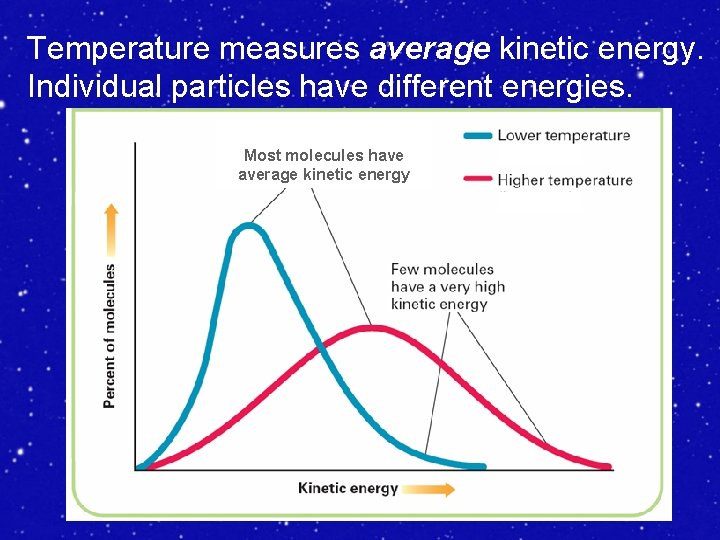Temperature measures average kinetic energy. Individual particles have different energies. Most molecules have average kinetic energySummary 8 § According to the graph: Which temperature shows the highest average kinetic energy?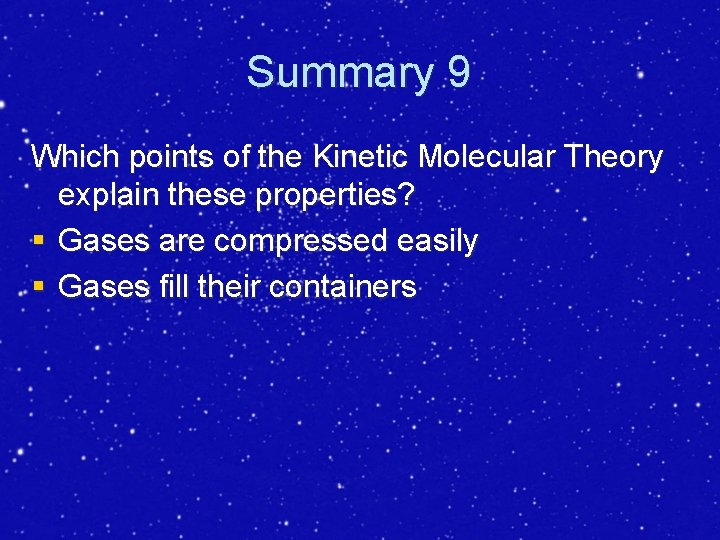Summary 9 Which points of the Kinetic Molecular Theory explain these properties? § Gases are compressed easily § Gases fill their containers4 b: Diffusion = ability of gases to mix § Particles move constantly § Particles are not attracted to each other Therefore: § Particles fill a container § Particles form a homogeneous mixture § § Lighter gases diffuse more quickly Heavy gases diffuse more slowlySummary 10 § What is a homogeneous mixture? Summary 11 § Helium gas and oxygen gas are released into a room at the same time. Which gas fills the room first? Explain why.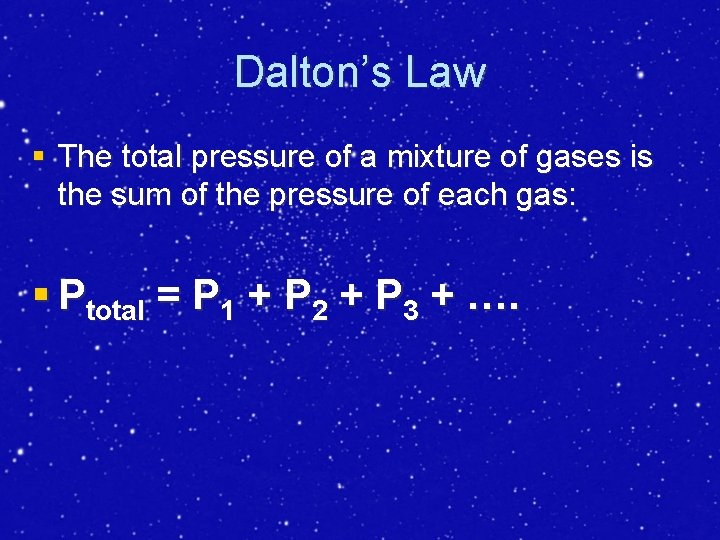Dalton’s Law § The total pressure of a mixture of gases is the sum of the pressure of each gas: § Ptotal = P 1 + P 2 + P 3 + ….Summary 12 At the summit of mount Everest the total pressure is about 0. 3 atm. § Assume PTotal = PN 2 + PO 2 § If PN 2 = 0. 25 atm what is the pressure of oxygen?4 c: The Gas Laws The combined gas law: for changing conditions P 1 V 1 = P 2 V 2 T 1 T 2 Initial conditions P 1 V 1 Initial pressure (atm) T 1 Initial temperature* (K) Initial volume (L) Final conditions P 2 V 2 Final pressure (atm) T 2 Final temperature* (K) Final volume (L) *Temperatures must be in Kelvin only§ Example: a gas at STP has a volume of 10 L. The pressure is increased to 5 atm. Temperature remains constant. What is the new volume? Initial conditions P 1 1 atm Final conditions P 2 5 atm V 1 10 L V 2 ? T 1 273 K T 2 = T 1 P 1 V 1 = P 2 V 2 T 1 T 2 P 1 V 1 T 2 = P 2 V 2 T 2 T 1 P 2 T 2 P 2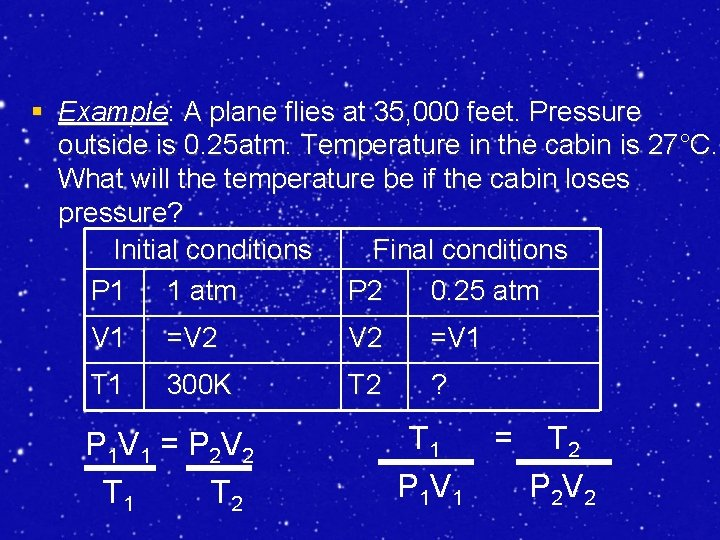§ Example: A plane flies at 35, 000 feet. Pressure outside is 0. 25 atm. Temperature in the cabin is 27°C. What will the temperature be if the cabin loses pressure? Initial conditions Final conditions P 1 1 atm P 2 0. 25 atm V 1 =V 2 =V 1 T 1 300 K T 2 ? P 1 V 1 = P 2 V 2 T 1 T 2 T 1 = T 2 P 1 V 1 P 2 V 2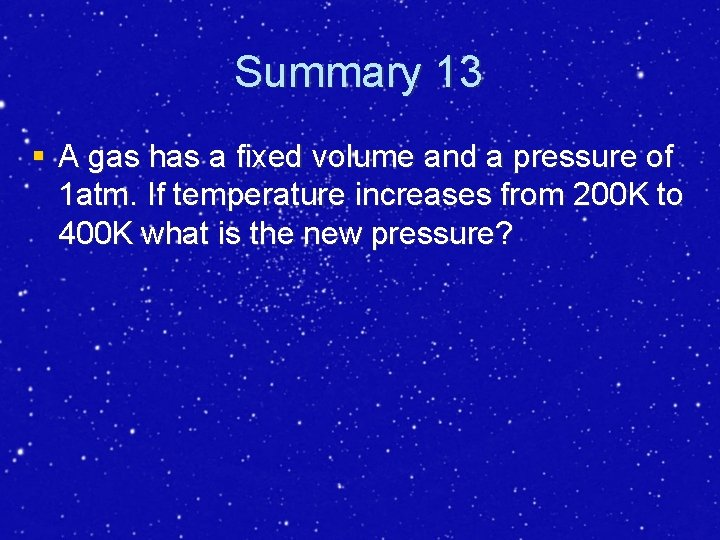Summary 13 § A gas has a fixed volume and a pressure of 1 atm. If temperature increases from 200 K to 400 K what is the new pressure?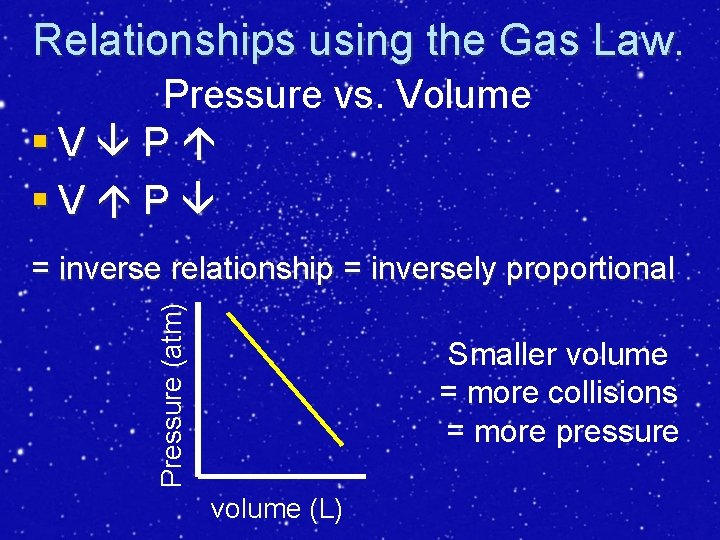Relationships using the Gas Law. Pressure vs. Volume §V P Pressure (atm) = inverse relationship = inversely proportional Smaller volume = more collisions = more pressure volume (L)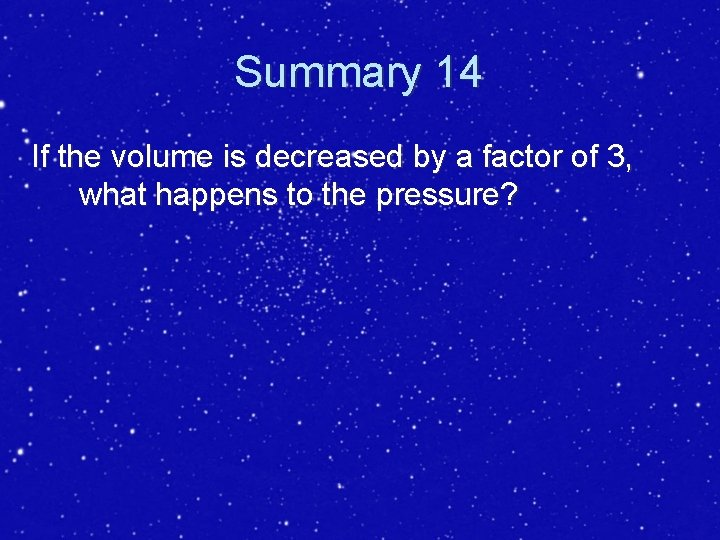Summary 14 If the volume is decreased by a factor of 3, what happens to the pressure?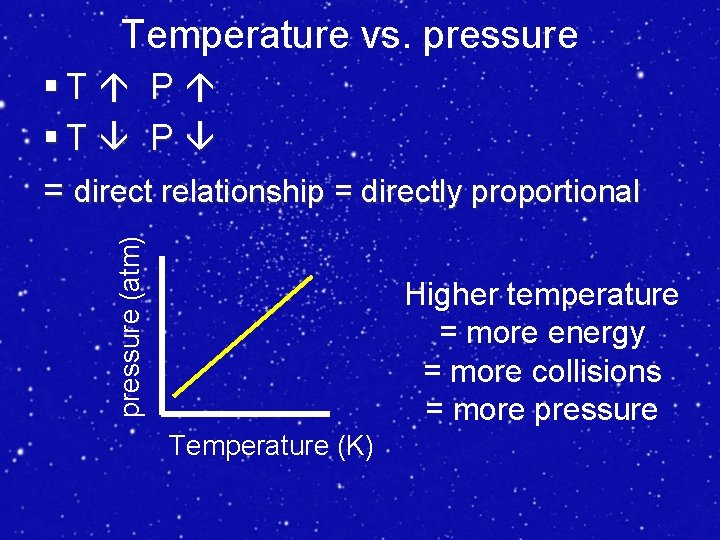Temperature vs. pressure (atm) §T P = direct relationship = directly proportional Higher temperature = more energy = more collisions = more pressure Temperature (K)Summary 15 If the temperature is tripled, what happens to the pressure?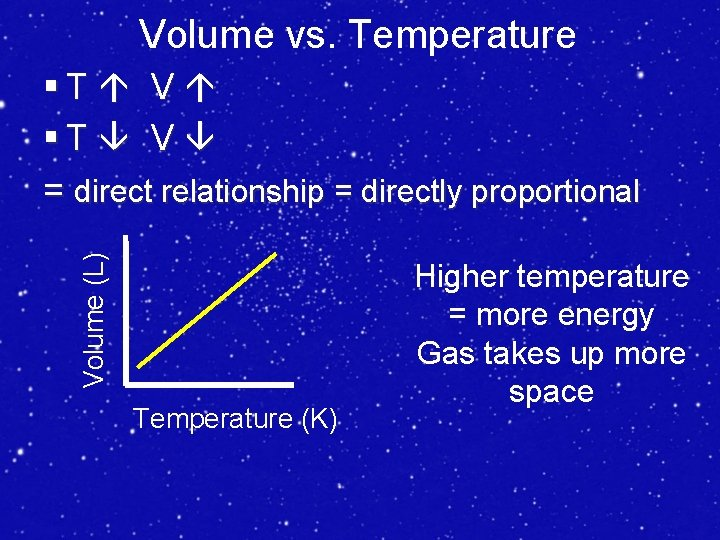Volume vs. Temperature Volume (L) §T V = direct relationship = directly proportional Temperature (K) Higher temperature = more energy Gas takes up more space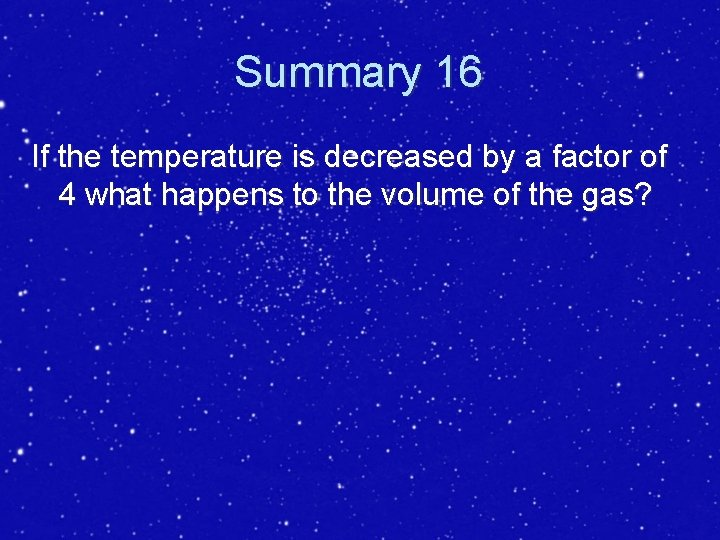Summary 16 If the temperature is decreased by a factor of 4 what happens to the volume of the gas?moles vs. pressure § n (=number of moles) P §n P = direct relationship = directly proportional More gas = more collisions = more pressure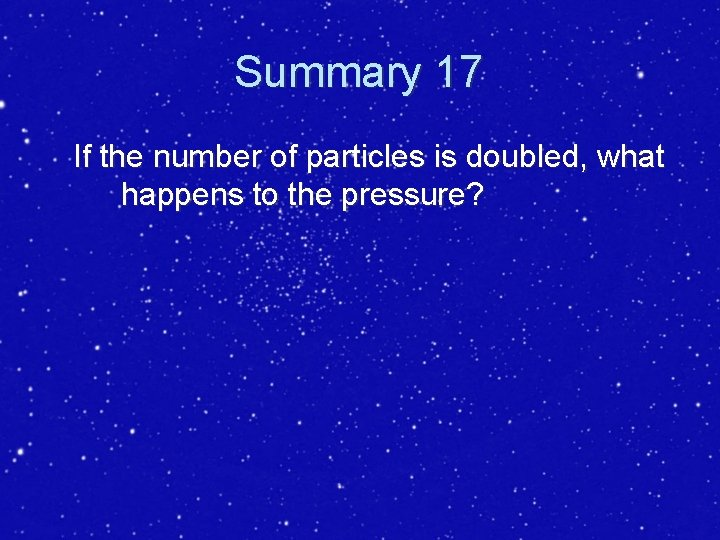Summary 17 If the number of particles is doubled, what happens to the pressure?Honors Only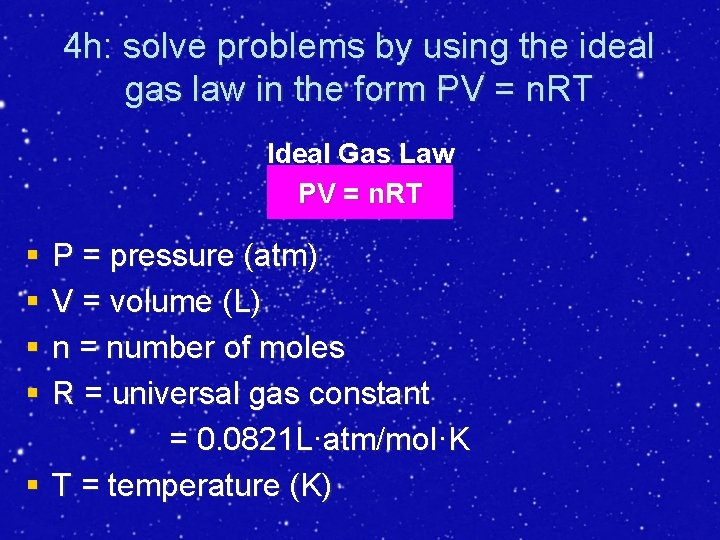4 h: solve problems by using the ideal gas law in the form PV = n. RT Ideal Gas Law PV = n. RT § § P = pressure (atm) V = volume (L) n = number of moles R = universal gas constant = 0. 0821 L·atm/mol·K § T = temperature (K)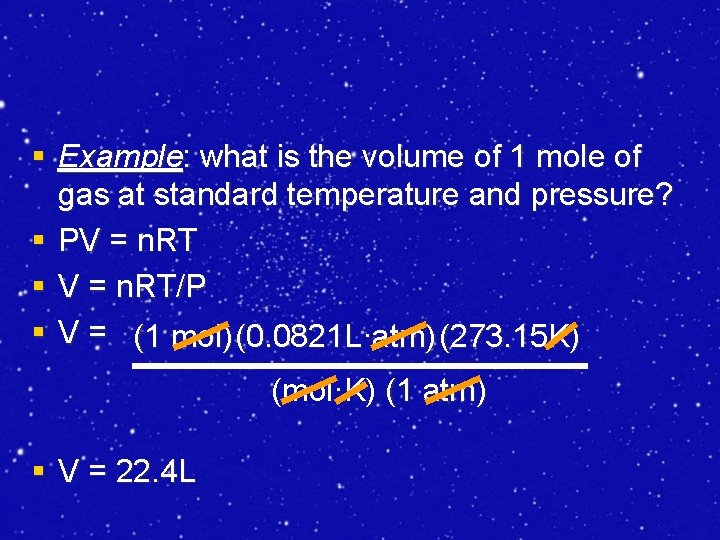§ Example: what is the volume of 1 mole of gas at standard temperature and pressure? § PV = n. RT § V = n. RT/P § V = (1 mol) (0. 0821 L·atm) (273. 15 K) (mol·K) (1 atm) § V = 22. 4 L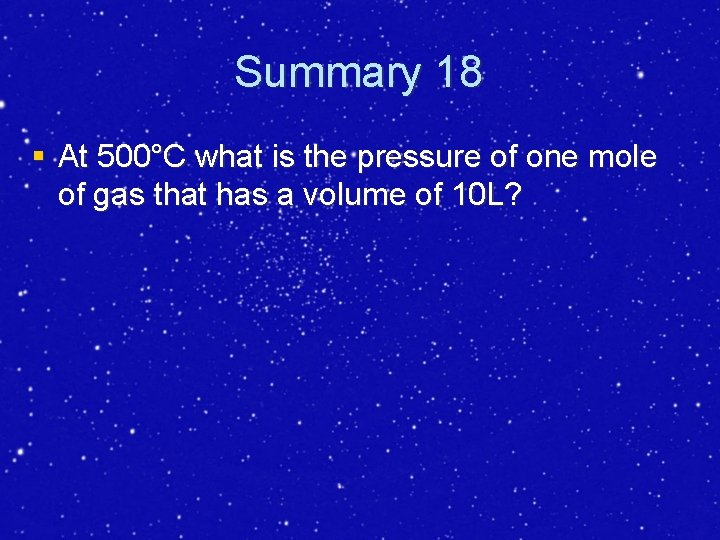Summary 18 § At 500°C what is the pressure of one mole of gas that has a volume of 10 L?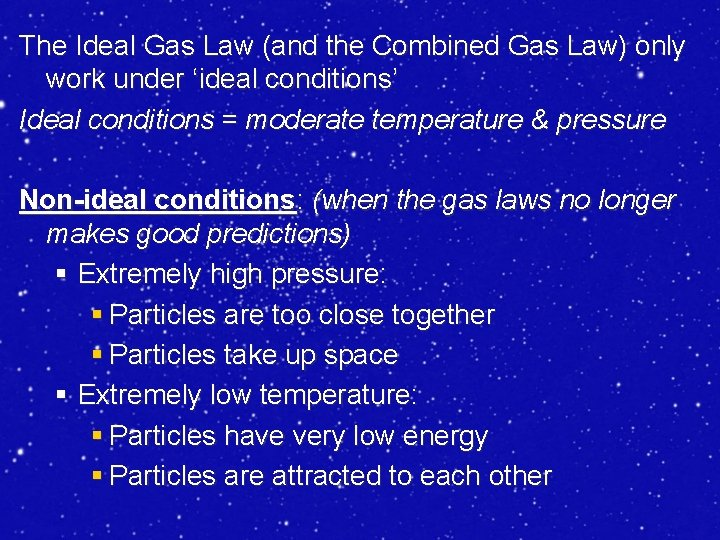The Ideal Gas Law (and the Combined Gas Law) only work under ‘ideal conditions’ Ideal conditions = moderate temperature & pressure Non-ideal conditions: (when the gas laws no longer makes good predictions) § Extremely high pressure: § Particles are too close together § Particles take up space § Extremely low temperature: § Particles have very low energy § Particles are attracted to each other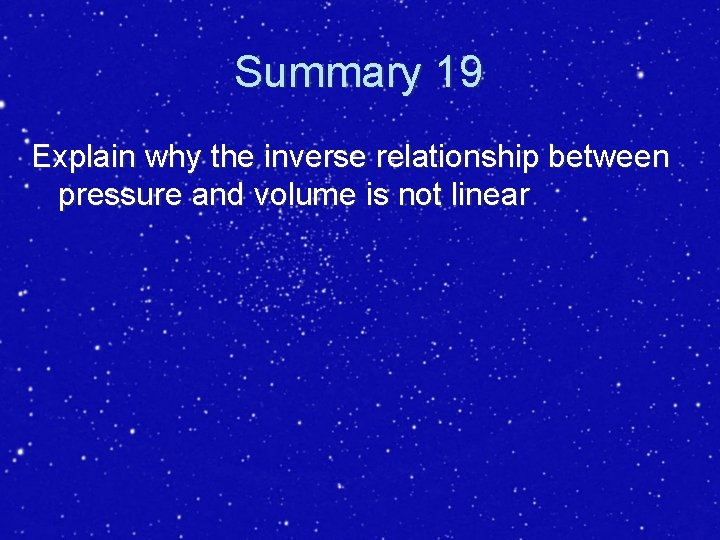Summary 19 Explain why the inverse relationship between pressure and volume is not linear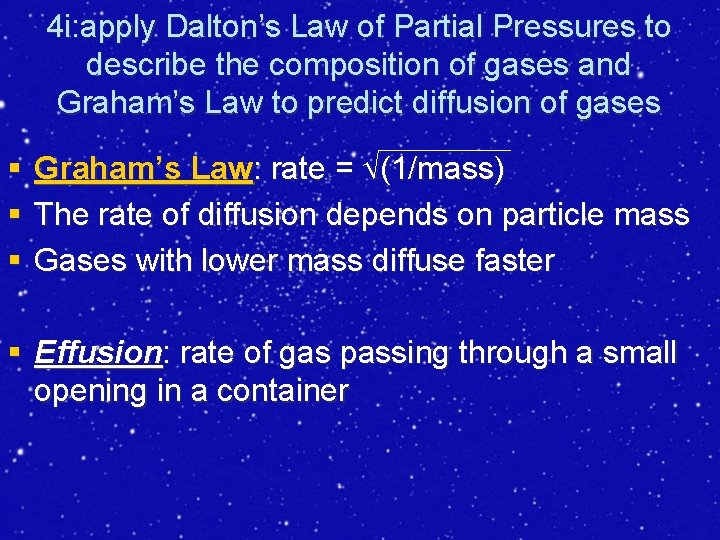4 i: apply Dalton’s Law of Partial Pressures to describe the composition of gases and Graham’s Law to predict diffusion of gases § § § Graham’s Law: rate = (1/mass) The rate of diffusion depends on particle mass Gases with lower mass diffuse faster § Effusion: rate of gas passing through a small opening in a containerSummary 20 § Which gas will effuse faster; chlorine or bromine? Why?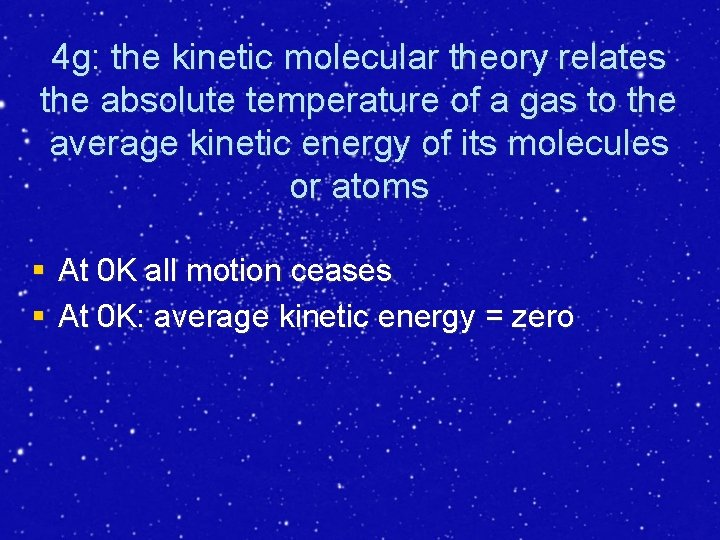4 g: the kinetic molecular theory relates the absolute temperature of a gas to the average kinetic energy of its molecules or atoms § At 0 K all motion ceases § At 0 K: average kinetic energy = zeroSummary 21 § If the average kinetic energy of a gas is zero, what is the temperature of that gas in Kelvin? Why?